### Home > CALC > Chapter Ch7 > Lesson 7.3.2 > Problem7-120

7-120.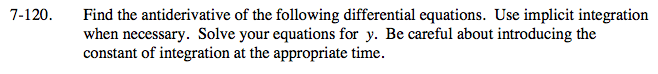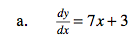Notice that the derivative is in terms of x. Antidifferentiate!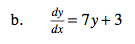Compare and contrast part (a) with part (b).
Which one requires implicit integration?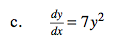Translation: The derivative is equal the the original function squared times a constant.
What can you conclude about the original function?
Is it linear, quadratic, cubic, exponential, trigonometric...?
Use implicit integration to check your conjecture.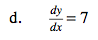Translation: The derivative of this function is a constant.
What can you conclude about the function?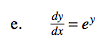y = −ln|−x + C|
Explain the significance of the absolute value.